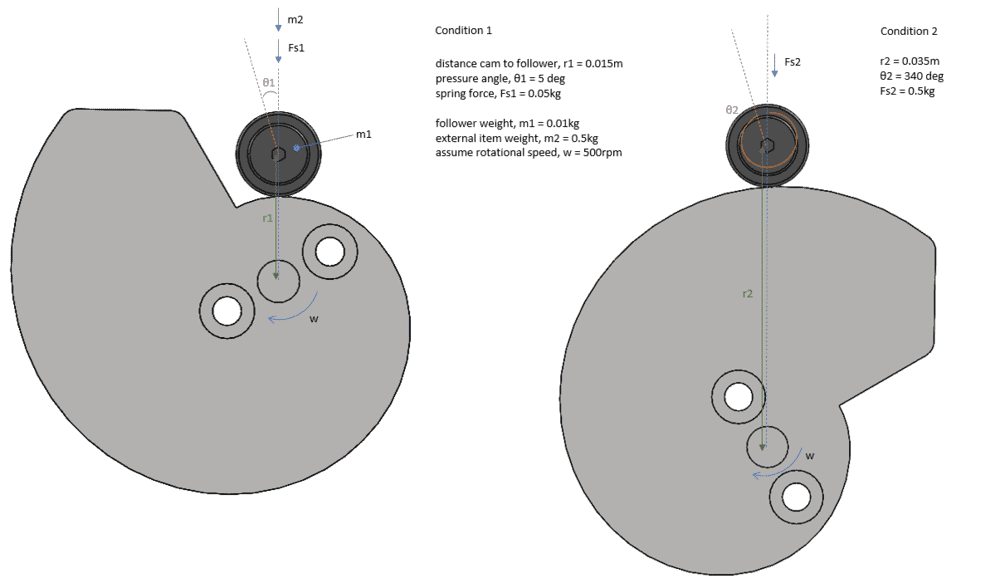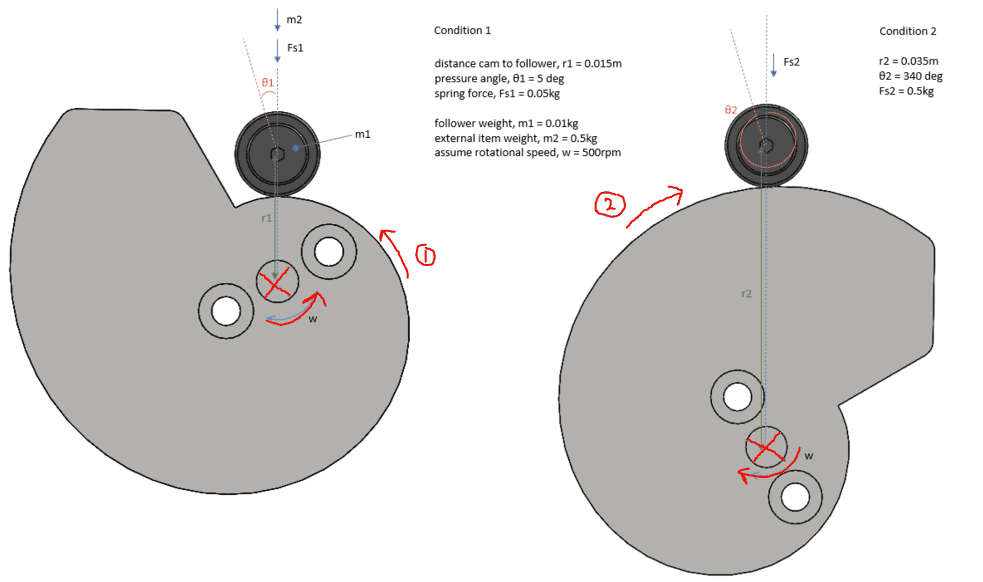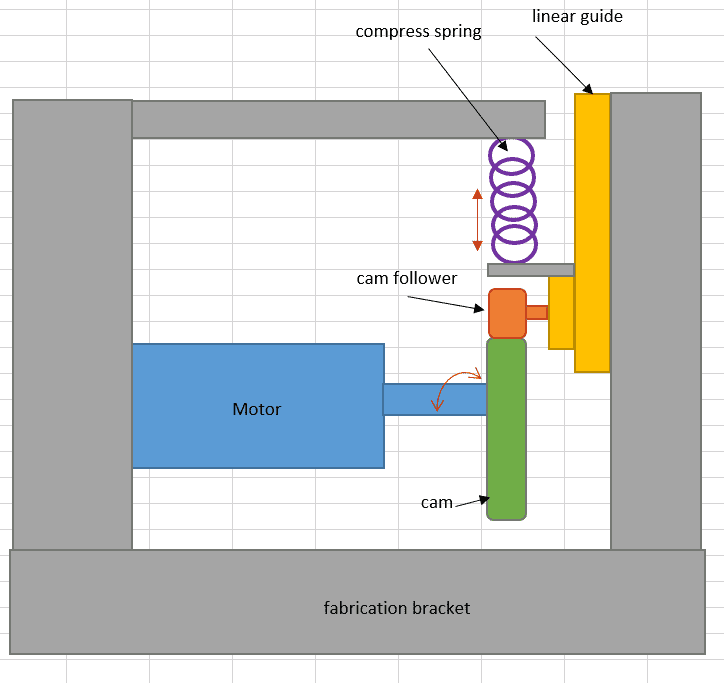# Calculate the required torque for a cam design

• Travis T
Travis T
TL;DR Summary
Would like to check the design of snail cam require how much motor rated torque?
Hi all,

I'm currently designing a cam mechanism.

Would like to check how much motor rated torque required for the following design of snail cam ?
To ease calculation, i have include some assumed factor as below. Two extreme condition (rotation degree) to compare.

What is the motor rated torque required for the design? (is 0.30Nm enough?)

*please let know if any factor missing or not logic.
*would like to have estimation for motor selection only, thus to simplify, insignificant and complicated factor can be ignoreMentor
Is this homework?

Calculate the torque at the extreme positions, at least three positions in between, and in each of the flat parts. Then decide if you need to calculate at some more points.

Show clearly the center of rotation, and the direction of rotation. Is the cam follower slowly rising, then falling fast? If so, calculate the fall time to find if it stays in contact with the cam.

Travis T
Is this homework?

Calculate the torque at the extreme positions, at least three positions in between, and in each of the flat parts. Then decide if you need to calculate at some more points.

Show clearly the center of rotation, and the direction of rotation. Is the cam follower slowly rising, then falling fast? If so, calculate the fall time to find if it stays in contact with the cam.

Hi Jr,

Thanks for reply, this is not a homework.

Dr.D
Which way does this turn? It will make a lot of difference.

Travis T
Which way does this turn? It will make a lot of difference.

Hi Dr. D,

condition1 shall be initial position,
when trigger, motor will rotate direction (1); cam follower will move up following the profile,
rotate until condition2, motor will stop (rev can be set; 340degree),
then motor rotate in reverse direction (2); cam follower back to initial position.Dr.D
Your problem statement is incomplete. What is there to resist motion? If these two elements were simply free in space (no gravity), they would be able to rotate without any applied torque at all. What resists the motion to require a torque?

Travis T
Your problem statement is incomplete. What is there to resist motion? If these two elements were simply free in space (no gravity), they would be able to rotate without any applied torque at all. What resists the motion to require a torque?

Hi Dr.D,

Compress spring is to always attach the cam follower to the profile of cam.
Here i attach a figure (side view) with other element for better understanding on the design.
Hope this could help explain the mechanism and question. Thanks.Dr.D
Ah, now you tell us! I presume you are aware that there are other ways to keep a follower in contact with a cam profile?

Do you know how to use the method of virtual work with a potential energy term included? That will get the system torque pretty easily (provided, of course that you specify the cam lift profile).

Travis T
Ah, now you tell us! I presume you are aware that there are other ways to keep a follower in contact with a cam profile?

Do you know how to use the method of virtual work with a potential energy term included? That will get the system torque pretty easily (provided, of course that you specify the cam lift profile).

Hi Dr.D,

No, i don't know for both ways and method. Would be great if you could give some advice on these.

I was thinking i can estimate the required motor torque by calculating resultant force from Condition2 (which is the highest torque required to reach the position moving from initial Condition1). Am i going the wrong direction?

Dr.D
Until you specify the cam lift curve, all you have is a picture. It is very hard (actually impossible) to apply mathematics to a picture. I suggest you get a machine design book (Shigley comes to mind) and read up on cam design.

•Travis T